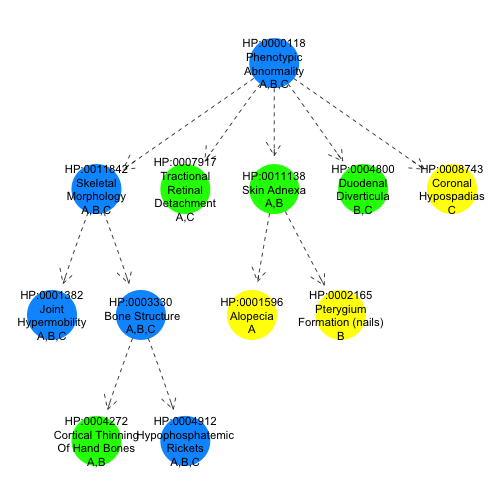hpoPlot lets you to represent sets of HPO terms (or indeed, sets of HPO encoded phenotypes each comprising one or more terms) by directed acyclic graphs corresponding to subgraphs of the HPO. The function provided for doing this is `hpo.plot`. To call it, you need to pass it the object which stores the structure of the HPO - `hpo.terms` which can be loaded with a call to `data`:

``````suppressPackageStartupMessages(library(hpoPlot))
data(hpo.terms)
``````

## Plotting Terms

A set of terms (character vector of HPO term codes) can be plotted as a DAG by calling hpo.plot:

``````hpo.plot(hpo.terms, terms=c("HP:0001873", "HP:0011877"))
``````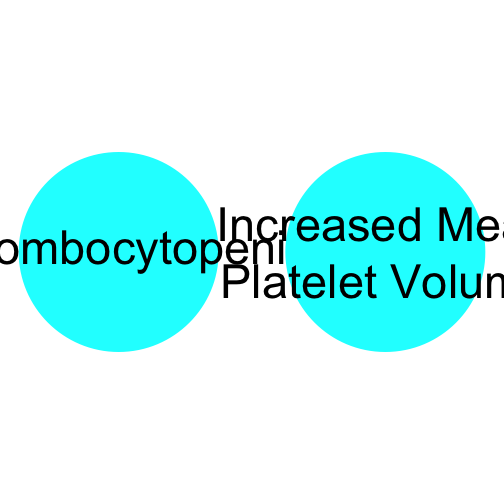To ensure all of the ancestor terms (according to the DAG structure of the HPO) are shown, we must first call `get.ancestors` on `terms`:

``````hpo.plot(hpo.terms, terms=get.ancestors(hpo.terms, c("HP:0001873", "HP:0011877")))
``````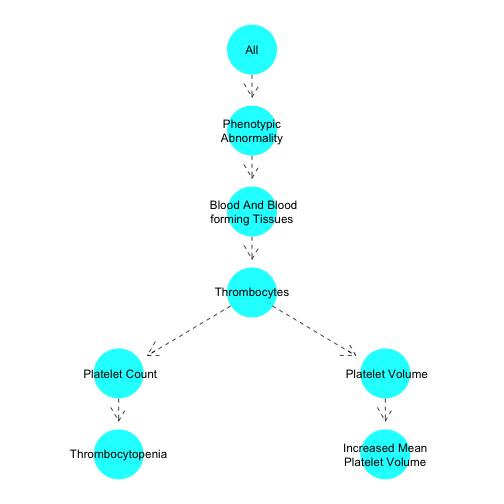Terms which just link a parent to a child term can be removed to save space, whilst retaining the structure of the relevant part of the ontology, with `remove.links`:

``````hpo.plot(hpo.terms, terms=remove.links(hpo.terms, get.ancestors(hpo.terms, c("HP:0001873", "HP:0011877"))))
``````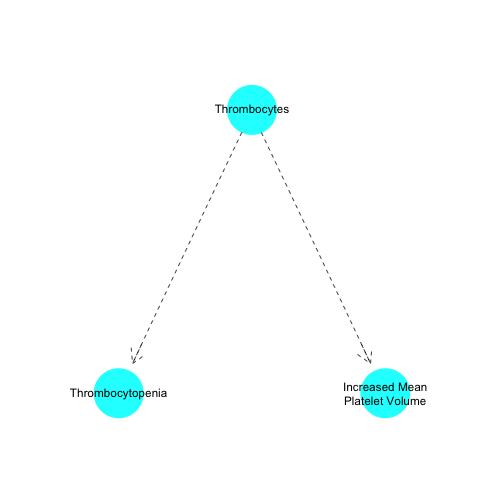Custom colours and labels can be passed to hpo.plot:

``````terms <- remove.links(hpo.terms, get.ancestors(hpo.terms, c("HP:0001873", "HP:0011877")))
hpo.plot(hpo.terms, terms=terms, colours=rainbow(length(terms)), labels=terms)
``````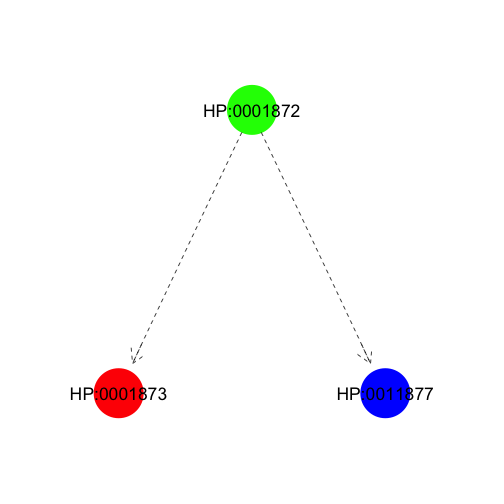Functions can be passed to hpo.plot which automatically select graphical parameters, e.g. the labels:

``````hpo.plot(hpo.terms, terms=terms, labels=get.code.node.labels)
``````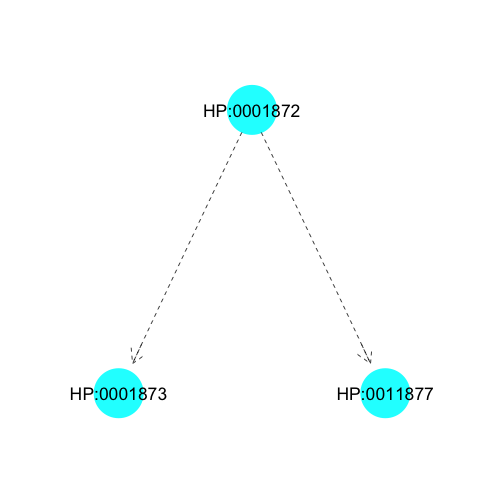## Plotting context

A `plotting.context` argument can be passed to the function hpo.plot. The argument should be a list of arguments which can be made use of by colouring/labelling functions. Examples include the frequency of nodes in a given population.

``````plotting.context <- list(frequency=seq(from=0, to=1, by=1/length(terms)))
names(plotting.context\$frequency) <- terms
hpo.plot(hpo.terms, terms=terms, plotting.context=plotting.context,
labels=get.code.node.labels, colours=get.pop.frequency.based.colours)
``````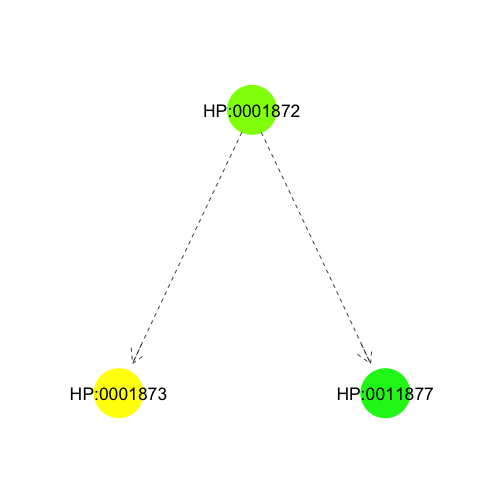Another useful argument to pass through `plotting.context` is a list of HPO encoded phenotypes (if this is passed, the `terms` argument of hpo.plot defaults to all the terms which are present either explicitly or implicitly (through the HPO) in the list of phenotypes). In the example below, the phenotypes are named A to C.

``````phenotype.strings <- c(
A="HP:0001382,HP:0004272,HP:0007917,HP:0004912,HP:0001596",
B="HP:0001382,HP:0004272,HP:0002165,HP:0004800,HP:0004912",
C="HP:0004800,HP:0001382,HP:0004912,HP:0007917,HP:0008743"
)

hpo.phenotypes <- term.set.list.from.character(phenotype.strings)

hpo.plot(hpo.terms, plotting.context=list(hpo.phenotypes=hpo.phenotypes), labels=get.case.based.labels)
``````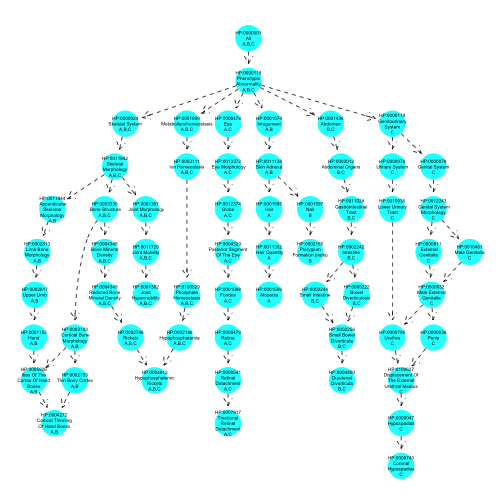Other features of the displayed nodes can be tuned with arguments to hpo.plot - these include, size, shape, font sizes, border colour (see `?hpo.plot` for details)

## Examples

If for a particular `plotting.context` there are many phenotypes to display, each with potentially a lot of terms, you can reduce the number of terms in the plot by removing those for which there is a more specific (i.e. 'descendant' in terms of the DAG structure of the HPO) term which is shared by exactly the same cases. The function which achieves this is called remove.uninformative.terms', and can be used as follows:

``````reduced.terms <- remove.uninformative.terms(hpo.terms, hpo.phenotypes)

hpo.plot(hpo.terms, plotting.context=list(hpo.phenotypes=hpo.phenotypes),
terms=reduced.terms,
labels=get.case.based.labels)
``````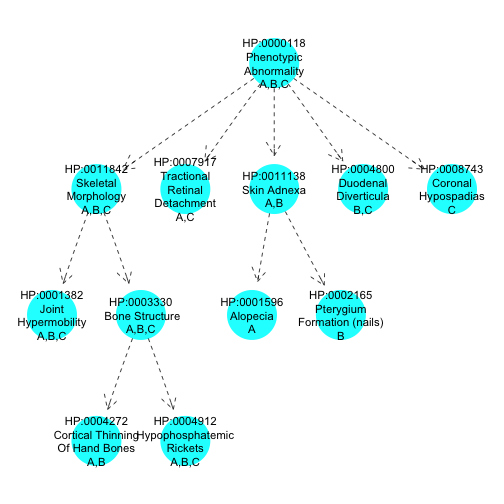To elaborate the plot further, we can use the frequency of each term in `hpo.phenotypes` them to colour them.

``````plotting.context <- list(frequency=get.term.frequencies(hpo.terms, hpo.phenotypes),
hpo.phenotypes=hpo.phenotypes)

hpo.plot(hpo.terms, plotting.context=list(hpo.phenotypes=hpo.phenotypes),
terms=reduced.terms,
labels=get.case.based.labels,
colours=get.frequency.based.colours)
``````